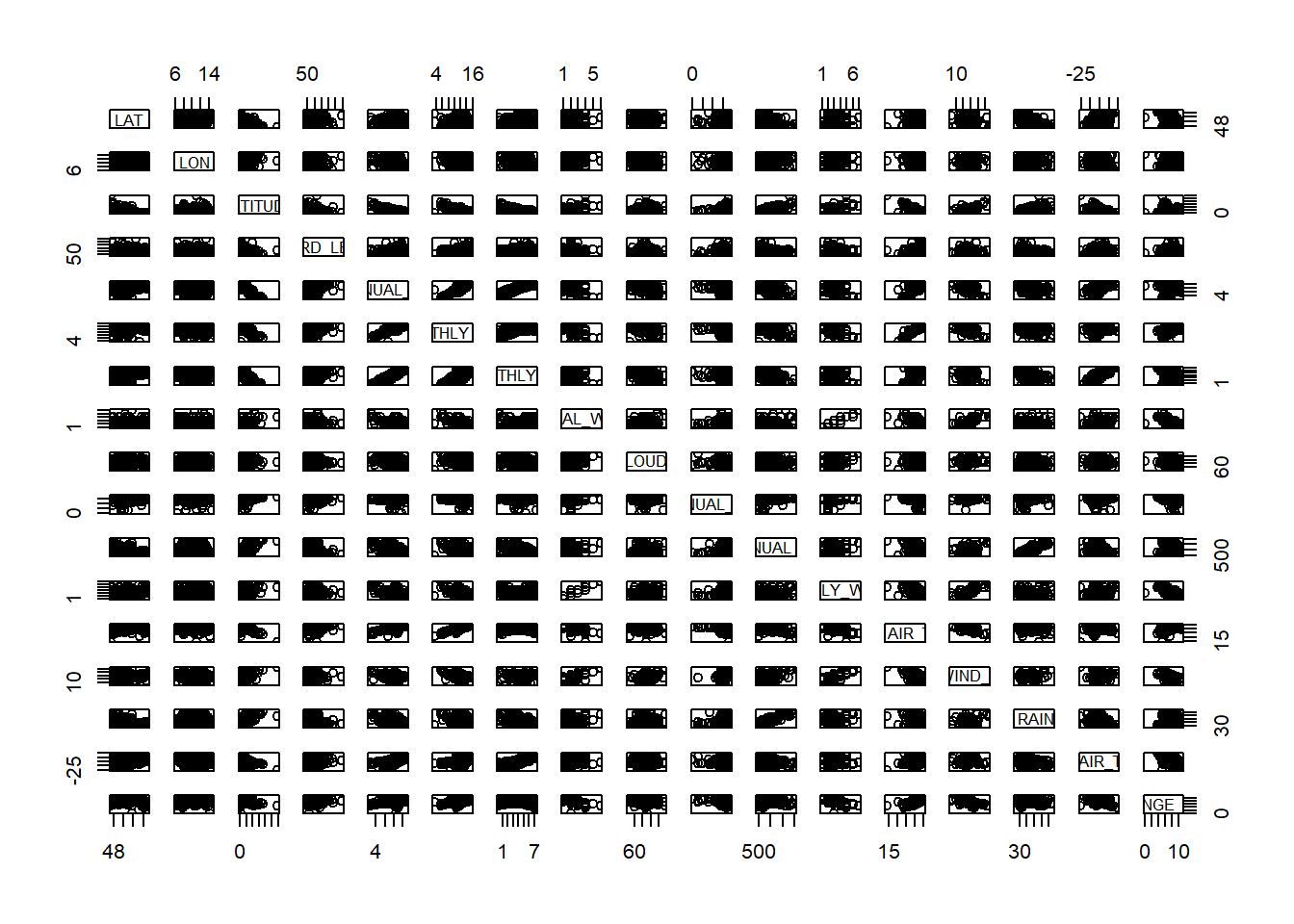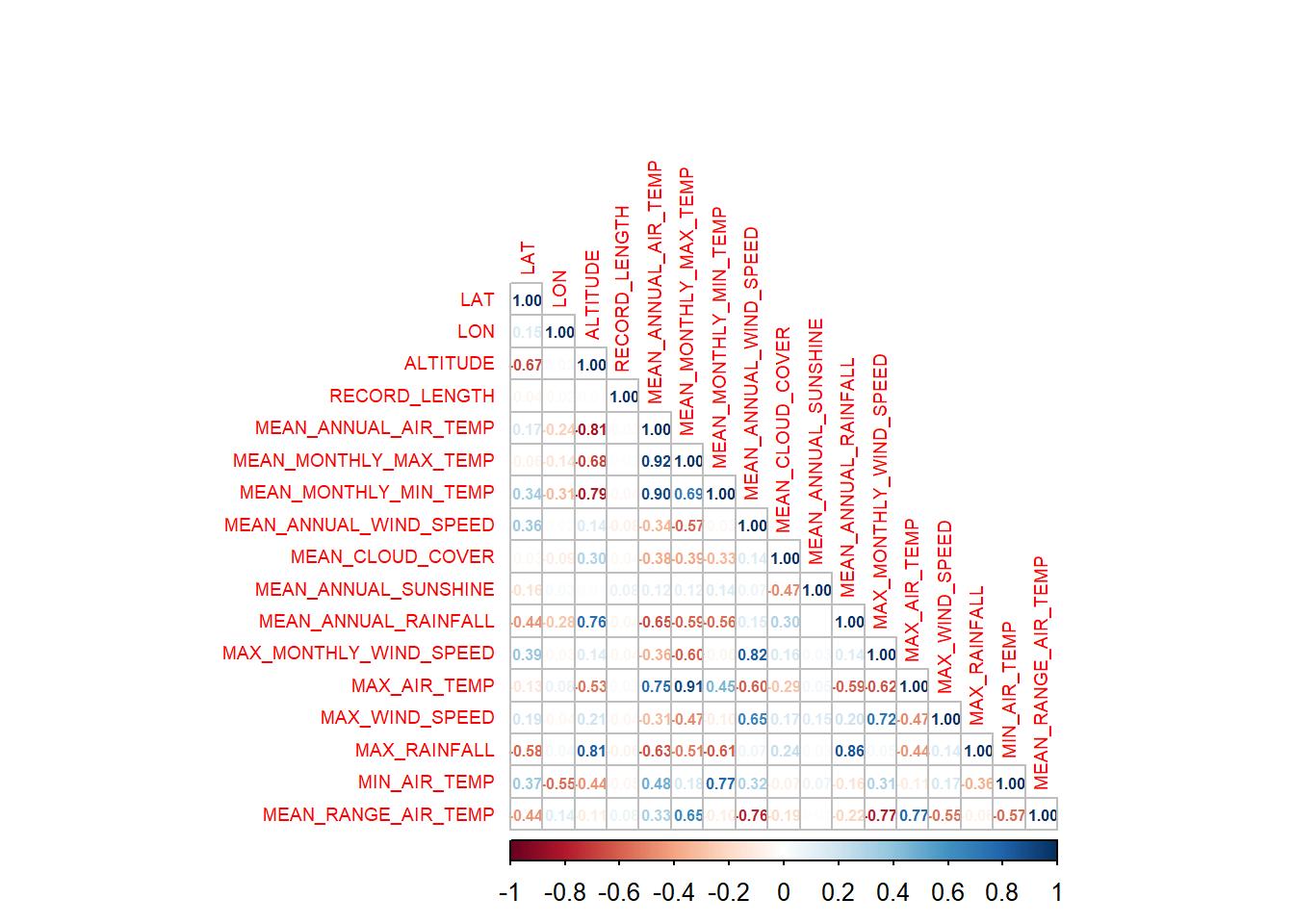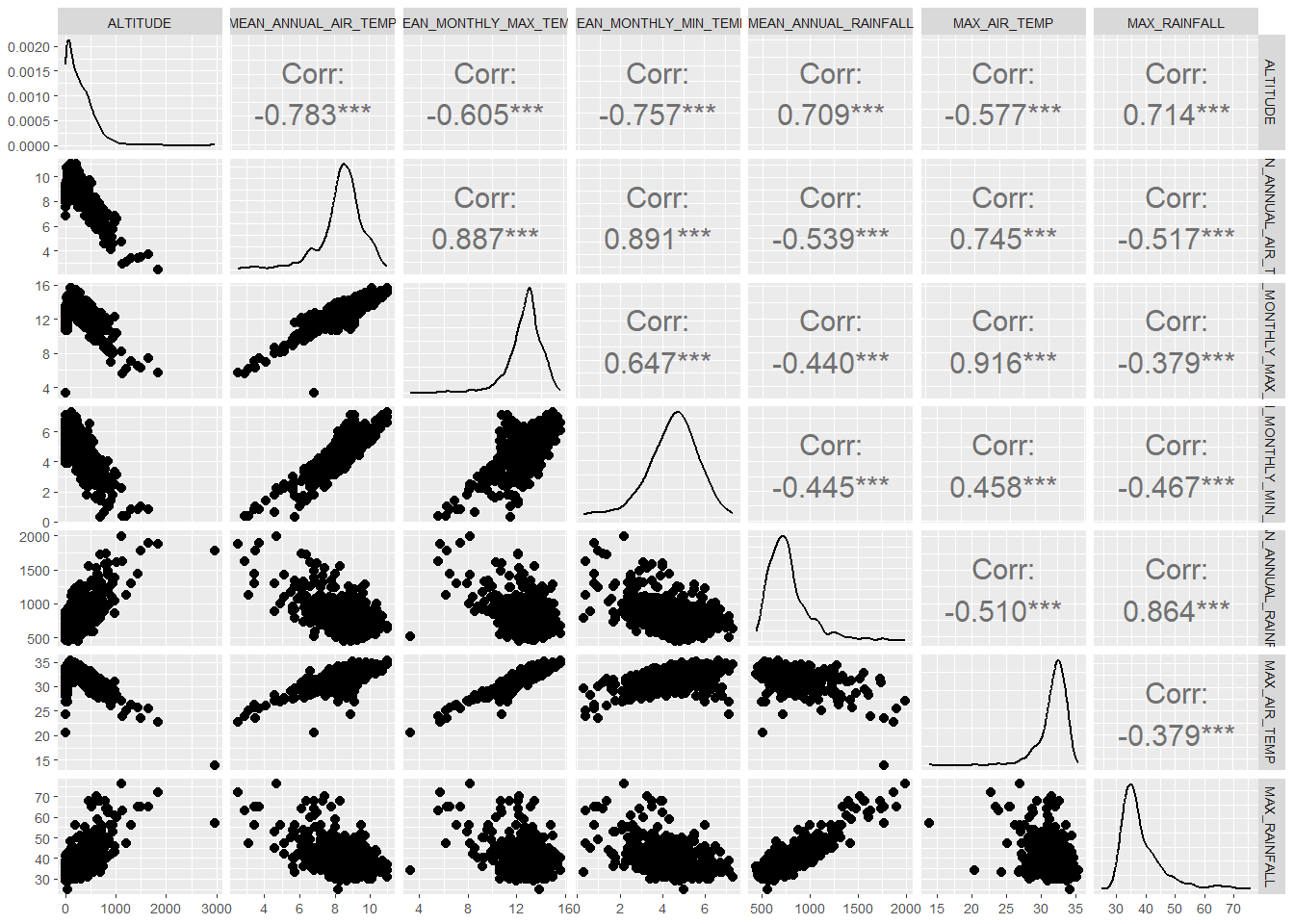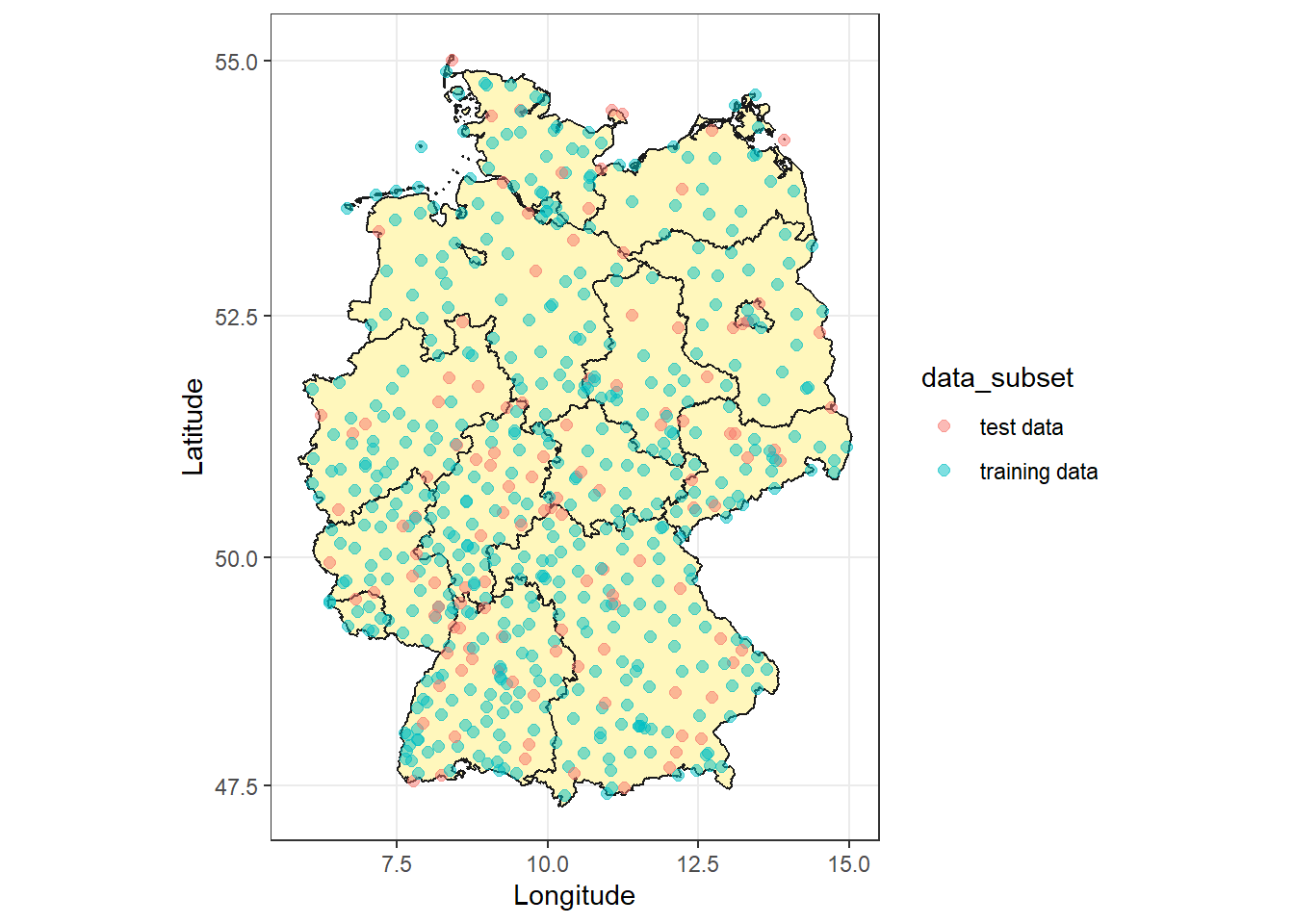So far we focused on an example data set with just two explanatory variables. In order to get some hands-on experience we advance by using a data set with more than two explanatory variables.

In order to showcase the multiple linear regression analysis we examine the relationship between the response variable MEAN.ANNUAL.RAINFALL and the other variables in the dwd data set.

You may download the DWD.csv file here. Import the data set and assign a proper name to it. Note that we use the read.csv2() function to load the data set. The read.csv() and read.csv2() are identical except for the defaults. The read.csv2() function is suitable for data originating from countries that use a comma as decimal point and a semicolon as field separator.

dwd <- read.csv2("https://userpage.fu-berlin.de/soga/data/raw-data/DWD.csv",
stringsAsFactors = FALSE, sep = ",")

The dwd data set consists of 599 rows, each of them representing a particular weather station in Germany, and 22 columns, each of them corresponding to a variable/feature related to that particular weather station. These self-explaining variables are: ID, DWD_ID, STATION_NAME, FEDERAL_STATE, LAT, LON, ALTITUDE, PERIOD, RECORD_LENGTH, MEAN_ANNUAL_AIR_TEMP, MEAN_MONTHLY_MAX_TEMP, MEAN_MONTHLY_MIN_TEMP, MEAN_ANNUAL_WIND_SPEED, MEAN_CLOUD_COVER, MEAN_ANNUAL_SUNSHINE, MEAN_ANNUAL_RAINFALL, MAX_MONTHLY_WIND_SPEED, MAX_AIR_TEMP, MAX_WIND_SPEED, MAX_RAINFALL, MIN_AIR_TEMP, MEAN_RANGE_AIR_TEMP.

The data was downloaded from the DWD (German Weather Service) data portal on April 21, 2017. You may find a detailed description of the data set here. Please note that for the purpose of this tutorial the data set was preprocessed and columns have been renamed.

#### Data preparation

Before we dive into the modelling we first have to prepare our data set. By calling the str() function on the data set we realize that there are features in the data set such as, id, DWD_ID, and STATION.NAME, among others, which we know, or at least presumably know, do not carry any particular useful information for the prediction of the mean annual rainfall. Thus, we exclude them from the data set. In addition we make sure that we exclude observations that contain missing values (NA).

str(dwd)
## 'data.frame':    599 obs. of  22 variables:
##  $ID : int 0 1 2 6 8 9 10 12 14 18 ... ##$ DWD_ID                : int  1 3 44 71 73 78 91 98 116 132 ...
##  $STATION_NAME : chr "Aach" "Aachen" "Großenkneten" "Albstadt-Badkap" ... ##$ FEDERAL_STATE         : chr  "Baden-Württemberg" "Nordrhein-Westfalen" "Niedersachsen" "Baden-Württemberg" ...
##  $LAT : chr "47.8413" "50.7827" "52.9335" "48.2156" ... ##$ LON                   : chr  "8.8493" "6.0941" "8.237" "8.9784" ...
##  $ALTITUDE : chr "478.0" "202.0" "44.0" "759.0" ... ##$ PERIOD                : chr  "1931-1986" "1851-2011" "1971-2016" "1986-2016" ...
##  $RECORD_LENGTH : int 55 160 45 30 64 55 38 67 67 33 ... ##$ MEAN_ANNUAL_AIR_TEMP  : chr  "8.2" "9.8" "9.2" "7.4" ...
##  $MEAN_MONTHLY_MAX_TEMP : chr "13.1" "13.6" "13.2" "12.2" ... ##$ MEAN_MONTHLY_MIN_TEMP : chr  "3.5" "6.3" "5.4" "3.3" ...
##  $MEAN_ANNUAL_WIND_SPEED: chr "2.0" "3.0" "2.0" "2.0" ... ##$ MEAN_CLOUD_COVER      : chr  "67.0" "67.0" "67.0" "66.0" ...
##  $MEAN_ANNUAL_SUNSHINE : chr "" "1531.0" "1459.0" "1725.0" ... ##$ MEAN_ANNUAL_RAINFALL  : chr  "755.0" "820.0" "759.0" "919.0" ...
##  $MAX_MONTHLY_WIND_SPEED: chr "2.0" "3.0" "3.0" "2.0" ... ##$ MAX_AIR_TEMP          : chr  "32.5" "32.3" "32.4" "30.2" ...
##  $MAX_WIND_SPEED : chr "" "30.2" "29.9" "" ... ##$ MAX_RAINFALL          : chr  "39.0" "36.0" "32.0" "43.0" ...
##  $MIN_AIR_TEMP : chr "-16.3" "-10.9" "-12.6" "-15.5" ... ##$ MEAN_RANGE_AIR_TEMP   : chr  "9.6" "7.3" "7.8" "8.9" ...
# Exclude variables
cols_to_drop <- c("ID",
"DWD_ID",
"STATION_NAME",
"FEDERAL_STATE",
"PERIOD") # columns to drop

rows_to_drop <- complete.cases(dwd) # rows to drop
dwd_data <- dwd[rows_to_drop, !(colnames(dwd) %in% cols_to_drop)]

After the data cleaning steps there are 599 rows (observations) and 17 columns (features) left in our data set.

dim(dwd_data)
##  599  17

Moreover, we split our data set at random into two distinct parts. One part is our training set while the other part will serve as our test set. The basic idea behind this data grouping strategy is, that we learn the model parameters on the training set, and then we use the test data set, a data set that was not involved at all during parameter estimation, to determine the goodness of fit of our model, or in other words by using two distinct data sets we may evaluate the generalization properties of our model.

For the sub-setting of the data set we rely on the sample.split() function from the caTools package, which splits the data set into two sets (training and test set) in a predefined ratio. Finally, we assign the response vector and the data matrix for each subset of the data a proper variable name for further processing.

# Split data into training and test set, 80% training and 20% test set
library(caTools)
## Warning: Paket 'caTools' wurde unter R Version 4.2.3 erstellt
set.seed(100) # set seed for reproducibility

# define split vector
split <- sample.split(dwd_data$MEAN_ANNUAL_RAINFALL, SplitRatio = 0.8) train_set <- dwd_data[split == TRUE, ] # training set test_set <- dwd_data[split == FALSE, ] # test set # response vectors y_train <- train_set$MEAN_ANNUAL_RAINFALL # response vector training set
y_test <- test_setMEAN_ANNUAL_RAINFALL # response vector test set # model matrices for training and test set # select all features except the response variable X_train <- as.matrix(subset(train_set, select = -c(MEAN_ANNUAL_RAINFALL))) X_test <- as.matrix(subset(test_set, select = -c(MEAN_ANNUAL_RAINFALL))) # save objects for later usage save(dwd, dwd_data, train_set, test_set, y_train, y_test, X_train, X_test, split, file = "dwd_30200.RData") Finally there remain 479 observations with 16 features in the data set to train our model, and 120 observations with 16 features to evaluate our model. #### Data visualization Before we build our model we visualize the data we are working with by plotting a scatter plot matrix. The R software environment provides several functions that allow us to plot scatter plot matrices. Just to name a few, we may use the pairs() function, the splom() function from the lattice library, the pairs.panels() function from the psych library or the ggpairs() function from the GGally library. The particular choice is a matter of experience, the size of the data set, and of personal taste. As a proof of concept that it becomes difficult to visualize a high dimensional feature space we apply the built-in function pairs() to the data set. dwd_data <- as.data.frame(sapply(dwd_data,as.numeric)) pairs(dwd_data)Arghhh! Such a plot is of no use at all! Note that we are just visualizing 17 features, which is compared to many other real world applications not much at all. A good strategy would be to choose only those pairs of variables that at least somehow are affecting our response variable MEAN.ANNUAL.RAINFALL. Thus, we first examine the pairwise correlation between the features, and then we select those features for visualization which show the highest correlation. The correlation of features can easily be calculated by using the cor() function, however, the output of cor() would still flood the screen, so that we do not improve our situation. A fancy method to overcome this issue is provided by the corrplot library. If we feed the eponymous corrplot() function with a correlation matrix we may visualize the correlations among the features in a quite convenient way. library(corrplot) dwd_data_cor <- cor(dwd_data, use = "complete.obs") corrplot(dwd_data_cor, method = "number", type = "lower", tl.cex = 0.6, number.cex = 0.5 )Take a look at the plot above and focus on the response variable MEAN.ANNUAL.RAINFALL at position (row and column) 11. In that particular row/column you find the Pearson correlation coefficients for each feature pair. The Pearson correlation coefficient is a number between $$-1$$ and $$+1$$. A positive number indicates a positive relation between the pairs of features and a negative number indicates a negative relation between the features, respectively. The more extreme the number the stronger the correlation. OK, back to our strategy: How to choose those pairs of variables that at least somehow are affecting our response variable MEAN.ANNUAL.RAINFALL? By now we have all the building blocks to solve this problem. We set a threshold level, let us say $$\pm 0.5$$. We select all feature pair combinations which have a correlation coefficient above or below that threshold, and then we plot them in a scatter plot matrix. Here we apply the ggpairs() function from the GGally library. This package is built upon the ggplot2 package and allows a lot of fine tuning. Check out the help page (type ?ggpairs in your console) for more information on the ggpairs() function. # set threshold cor_threshold <- 0.5 # extract feature names best_features <- row.names(dwd_data_cor)[abs(dwd_data_cor["MEAN_ANNUAL_RAINFALL", ]) > cor_threshold] print(best_features) ##  "ALTITUDE" "MEAN_ANNUAL_AIR_TEMP" "MEAN_MONTHLY_MAX_TEMP" ##  "MEAN_MONTHLY_MIN_TEMP" "MEAN_ANNUAL_RAINFALL" "MAX_AIR_TEMP" ##  "MAX_RAINFALL" library(ggplot2) library(GGally) # call ggpairs() ggpairs(dwd_data, columns = c(best_features), upper = list(continuous = wrap("cor", size = 4, alignPercent = 1) ) ) + theme_grey(base_size = 6.5)Finally, we get a nice and clear representation of a subset of features of the dwd data set, all of them showing some kind of a relation among each another. Obviously, all variables are more or less skewed by physical constraints. Thus, we should keep in mind, that our results may be biased by missing normality and should be transformed prior to further analyses. For the sake of straightforwardness, we will ignore this important point here. In the next section we build a bunch of multiple linear regression models and we are going to investigate these relationships in more detail. However, before we continue we should remind ourselves that the data set we are working with has a spatial component. Basically, our observations are point measurements of meteorological parameters spread across Germany. Let us plot a simple map to get a feel of the spatial distribution of our observations. Therefore we rely on the raster package and on the ggplot2 package. library(raster) library(ggplot2) # retrieve federal states by the getData() function from the raster package G1 <- getData(country = "Germany", level = 1) # add a new column called "data_subset" to the data set and populate the column with a character string of "training data" or "test data" based on the split variable from the section DATA PREPARATION dwd_datadata_subset <- ifelse(split == TRUE, "training data", "test data")

# plot the map
library(mapproj)
ggplot() +
geom_polygon(data = G1,
aes(x = long, y = lat, group = group),
colour = "grey10", fill = "#fff7bc") +
geom_point(data = dwd_data,
aes(x = LON, y = LAT, colour = data_subset),
alpha = .5,
size = 2) +
theme_bw() +
xlab("Longitude") + ylab("Latitude") +
coord_map()Nice! We realize that our data is quite well distributed across Germany. Further, it appears that the observations in the test data set are as well randomly distributed across the country.

Citation

The E-Learning project SOGA-R was developed at the Department of Earth Sciences by Kai Hartmann, Joachim Krois and Annette Rudolph. You can reach us via mail by soga[at]zedat.fu-berlin.de.You may use this project freely under the Creative Commons Attribution-ShareAlike 4.0 International License.

Please cite as follow: Hartmann, K., Krois, J., Rudolph, A. (2023): Statistics and Geodata Analysis using R (SOGA-R). Department of Earth Sciences, Freie Universitaet Berlin.